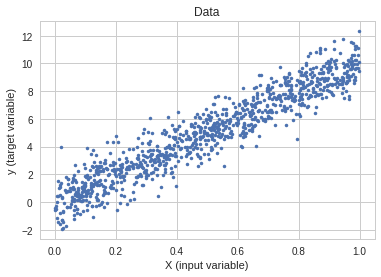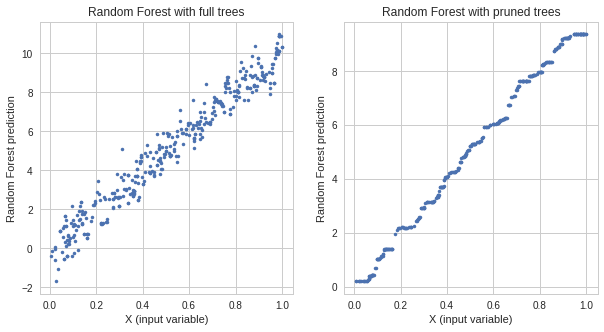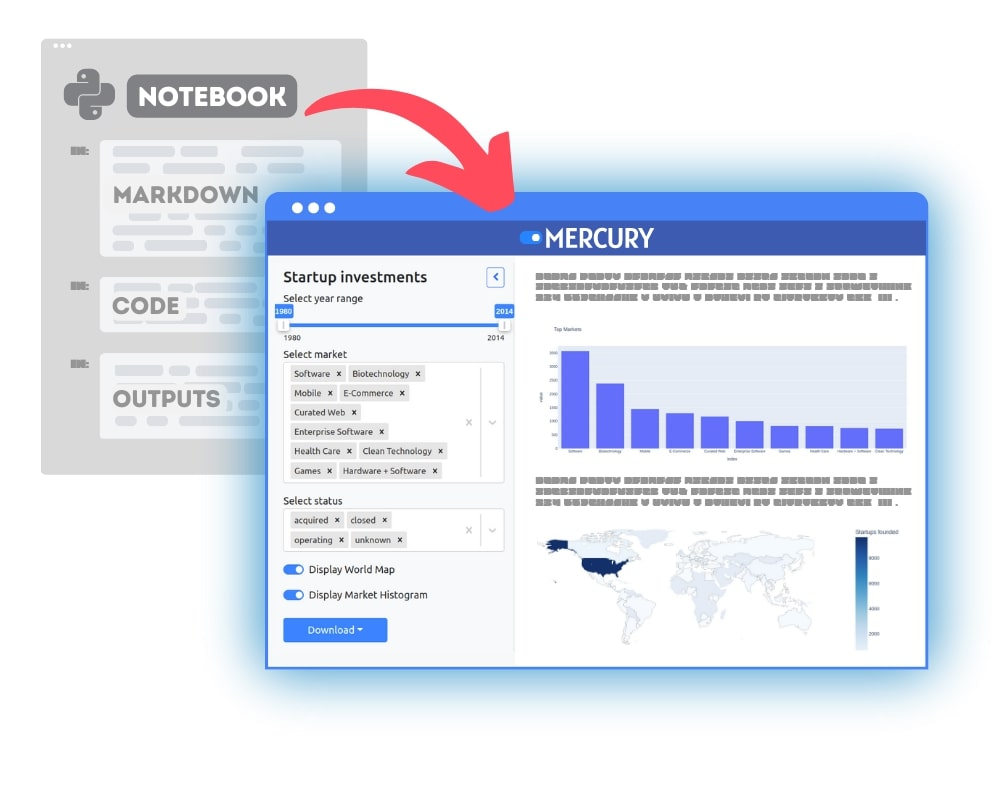When I first saw this question I was a little surprised. The first thought is, of course, they do! Any complex machine learning algorithm can overfit. I’ve trained hundreds of Random Forest (RF) models and many times observed they overfit. The second thought, wait, why people are asking such a question? Let’s dig more and do some research. After quick googling, I’ve found the following paragraph on Leo Breiman (the creator of the Random Forest algorithm) website:

Random forests does not overfit. You can run as many trees as you want.

The link to this statement on Breiman website. (BTW, on his website, the performance of RF was checked on 800MHz processor!)

What? Impossible! So RF doesn’t overfit? The developer of the algorithm knows the best - I thought. He is much smarter than me. For sure he is right. But wait, I’ve seen many times that RF was overfitting. Uh, I’m very confused!

Let’s try to check it, from a theoretical and practical point of view.

### Random Forest Theory

Random Forest is an ensemble of decision trees. The single decision tree is very sensitive to data variations. It can easily overfit to noise in the data. The Random Forest with only one tree will overfit to data as well because it is the same as a single decision tree. When we add trees to the Random Forest then the tendency to overfitting should decrease (thanks to bagging and random feature selection). However, the generalization error will not go to zero. The variance of generalization error will approach to zero with more trees added but the bias will not! It is a useful feature, which tells us that the more trees in the RF the better. And maybe that was the thought Breiman had when writing about RF and overfitting.

### The Random Forest overfitting example in python

To show an example of Random Forest overfitting, I will generate a very simple data with the following formula:

``````y = 10 * x + noise
``````

I will use `x` from a uniform distribution and range 0 to 1. The noise is added from a normal distribution with zero mean and unit variance to `y` variable. The plot of our data example is below.The code to generate the data and split it into a train and test subsets:

``````data = np.random.uniform(0, 1,(1000, 1))
noise = np.random.normal(size=(1000,))
X = data[:,:1]
y = 10.0*(data[:,0]) + noise
# split to train and test
X_train, X_test, y_train, y_test = sklearn.model_selection.train_test_split(X, y, test_size=0.4, random_state=2019)
``````

There is only one input variable `x` in our Random Forest model and one output variable `y`. To train the Random Forest I will use python and scikit-learn library. I will train two models one with full trees and one with pruning controlled by `min_samples_leaf` hyper-parameter. The code to train Random Forest with full trees:

``````rf = RandomForestRegressor(n_estimators=50)
rf.fit(X_train, y_train)
y_train_predicted = rf.predict(X_train)
y_test_predicted_full_trees = rf.predict(X_test)
mse_train = mean_squared_error(y_train, y_train_predicted)
mse_test = mean_squared_error(y_test, y_test_predicted_full_trees)
print("RF with full trees, Train MSE: {} Test MSE: {}".format(mse_train, mse_test))
``````

For error the Mean Squared Error was used - the lower the better. The RF with full trees get MSE: 0.20 on train data and MSE: 1.41 on test data. Let’s check RF with pruned trees:

``````rf = RandomForestRegressor(n_estimators=50, min_samples_leaf=25)
rf.fit(X_train, y_train)
y_train_predicted = rf.predict(X_train)
y_test_predicted_pruned_trees = rf.predict(X_test)
mse_train = mean_squared_error(y_train, y_train_predicted)
mse_test = mean_squared_error(y_test, y_test_predicted_pruned_trees)
print("RF with pruned trees, Train MSE: {} Test MSE: {}".format(mse_train, mse_test))
``````

For RF with pruned trees, the MSE on train data is 0.91 and MSE on a test is 1.04.

We have clear evidence of overfitting! The RF with full trees has a much lower error on train data than the RF with pruned trees but the error on test data is higher - the overfitting! Let’s visualize this on the scatter plot. On the left, there is a response from overfitted Random Forest and on the right the response of the Random Forest with pruned trees.We see the RF with full trees, which overfitted, predicts a noise which it learns during the training. The response from the RF with pruned trees is much smoother. Hence, its generalization is better.

### Overfitting and a growing number of trees

Let’s check how the Random Forest model will behave while growing number of trees. We will train the RF model starting with 1 tree and add 1 tree in each loop iteration. In every step, we will measure MSE on train and test data.

``````rf = RandomForestRegressor(n_estimators=1)
for iter in range(50):
rf.fit(X_train, y_train)
y_train_predicted = rf.predict(X_train)
y_test_predicted = rf.predict(X_test)
mse_train = mean_squared_error(y_train, y_train_predicted)
mse_test = mean_squared_error(y_test, y_test_predicted)
print("Iteration: {} Train mse: {} Test mse: {}".format(iter, mse_train, mse_test))
rf.n_estimators += 1
``````

When we plot the measurements we got:As you see we were using the RF with full trees, which we know that overfits the data. However, as you can observe from the plot. The overfitting does not increase by adding more trees to the RF model. It stabilizes with more trees.

## Summary

• The Random Forest algorithm does overfit.
• The generalization error variance is decreasing to zero in the Random Forest when more trees are added to the algorithm. However, the bias of the generalization does not change.
• To avoid overfitting in Random Forest the hyper-parameters of the algorithm should be tuned. For example the number of samples in the leaf.
• Here is a link to all code in Google Colab notebook.### Convert Python Notebooks to Web Apps

We are working on open-source framework Mercury for converting Jupyter Notebooks to interactive Web Applications.### Articles you might find interesing